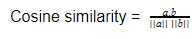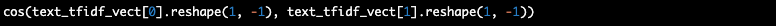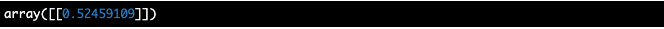Home## DETaiLED: Cosine Similarity, the metric behind recommendation systems

By Dhilip Subramanian

#### Highlights

There are many similarity metrics used in recommendation systems, and one of the most commonly used similarity metric - “Cosine similarity”.

Machine learning algorithms provide customized suggestions in our day-to-day life. For example, recommending products in e-commerce sites to recommending movies in movie streaming platforms or recommending a book to borrow from a public library based on your current or previous preferences.

Algorithms that are used to provide the list of suggestions are called recommendation systems or engines. The recommendation system in its core algorithm uses a fundamental mathematical metric called “similarity”, which compares and quantifies the similarity between two items: user selected vs rest of items in the catalog. The list of items with high similarity values to the ones that the user selected are recommended as “You may also like”.

There are many similarity metrics used in recommendation systems. Let us focus on the most commonly used similarity metric - “Cosine similarity”. We understand that this metric calculates and quantifies the similarity between two items, but how?

To understand the fundamentals, let us look into the example of two jet skis riding in a lake. Let’s say that both of the jet skis are riding in the north-east direction. That means, there are two features here (two-dimensional space): North and East. The jet ski position is represented by numerical values in both north and east direction and the position of the jet skis can be represented as vectors from the origin.Image inspired from here: Cosine similarity - Mastering Machine Learning with Spark 2.x [Book]

Now, to get the cosine similarity between the jet skis in the north-east dimensions, we need to find the cosine of the angle between these two vectors.

Cosine similarity = cos(item1, item2)

So, for case (a) in the figure, cosine similarity is,

Cosine similarity = cos(blue jet ski, orange jet ski) = cos(30°) = 0.866

Now to determine if Case(b) and Case(c) are similar to Case(a), we can apply cosine similarity to other two cases,

Case (b):

cos(0°) = 1 [Similar]

Case (c):

cos(90°) = 0 [Not similar]

Cosine similarity ranges from -1 to 1. 1 indicates the items are the same whereas -1 represents the compared items are dissimilar. Note that the cosine similarity in case (b) is 1 (similar) though the size of the blue jet ski vector is higher than the orange one. This indicates that cosine similarity is independent of the magnitude or the size of the vectors. It depends only on the direction of the vectors. When the angle between the vectors is small, the similarity is higher.

In practical applications where finding the angle between vectors is non-trivial, we can use the cosine formulation below obtained from the inner product between two vectors in linear algebra:where, a and b are the vectors of items a and b, and ||a|| and ||b|| are the Euclidean norm or the magnitude of the vector. This formula is more apt for machine learning applications, where we know the values of the vectors.

Let us see how cosine similarity is calculated in python. For simplicity, two simple documents are used as example: "I love pasta” and "I do not love pasta"To vectorize the documents, Term Frequency Inverse Document Frequency (TF-IDF) vectorizer with unigram and bigram is used. The vector notation of the two documents are:Now, the similarity between the two vectors as per cosine similarity can be calculated using Scikit-learn library’s built-in cosine_similarity function:The cosine similarity between the two documents is 0.5. We might wonder why the cosine similarity does not provide -1 (dissimilar) as the two documents are exactly opposite. This reminds us that cosine similarity is a simple mathematical formula which looks only at the numerical vectors to find the similarity between them. If we were to include more data and use additional methods for vectorization, we would get a “true” vector representation of these documents, which can then improve the similarity. The more accurate the vector representation of the real world object, more accurate is the cosine similarity between them.#### Dhilip Subramanian

Dhilip is Machine Learning Engineer working in Wellington, NZ and an AI enthusiast who is passionate with Data Science, Machine Learning and Data Visualization. He loves to explain AI concepts into a simpler term. He is a contributor to the SAS community and blogger in various data science platforms.

Image by Martin Pyško from Pixabay#### Previous Article

Punjab's Agri Export Corporation is using Al for improving the quality of seed potatoes